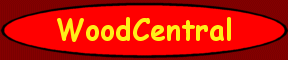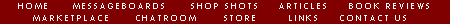ARTICLES & REVIEWS

The Golden Ratio/Fibonacci Series
or Charting the Populations of Rabbits(!)

### Excerpts from The Message Boards Assembled by Garrett Lambert

Louis McGrath asked, “having never seen a writeup on the Golden Rule for Dummies, I have a question that I can't answer on my own. Given a vertical measurement of 897/8" , divided into three different lengths, longest on the bottom, shortest at the top, what are the three lengths that fit into the golden rule? An explanation of how you came to your answer would be appreciated.

A number of very useful replies were posted—copied below—but none provided any background on the origin of the Rule. My curiosity aroused, I did a search and came across an interesting website that introduced the Rule's originator, a 12th century mathematician named Leonardo Fibonacci. Because I think others at WoodCentral will be equally surprised—and impressed—I contacted Karen Sadler at University of Arkansas who graciously gave permission for me to use the information which I have re-cast as follows:

Leonardo Fibonacci was born in Pisa, Italy, around 1175 and died there some time after 1240. His father was Guilielmo Bonacci, a secretary of the Republic of Pisa. His father was also a customs officer for the North African city of Bugia. Some time after 1192. Bonacci brought his son with him to Bugia. Guilielmo wanted Leonardo to become a merchant, and so arranged for his instruction in calculational techniques, especially those involving the Hindu–Arabic numerals which had not yet been introduced into Europe. Since Fibonacci was the son of a merchant, he was able go travel freely all over the Byzantine Empire. This allowed him to visit many of the area's centers of trade where he was able to learn both the mathematics of the scholars, and the calculating schemes in popular use at the time. He returned to Pisa around 1200 to become the greatest European mathematician of the Middle Ages. He was the first to introduce the Hindu–Arabic number system into Europe, and in 1202 completed a book on how to do arithmetic in the decimal system (titled Liber abaci. It describes the rules we all now learn in elementary school for adding, subtracting, multiplying and dividing).

Leonardo`s mathematical interests and abilities encompassed the practical as well as theoretical. A problem in the third section of Liber abaci led to the introduction of the Fibonacci numbers: "A certain man put a pair of rabbits in a place surrounded on all sides by a wall. How many pairs of rabbits can be produced from that pair in a year if it is supposed that every month each pair begets a new pair which from the second month on becomes productive?"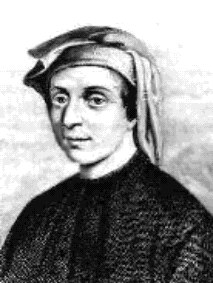By charting the populations of rabbits Fibonacci discovered a number series from which one can derive the Golden Section. Here`s the beginning of the sequence:

 1, 1, 2, 3, 5, 8, 13, 21, 34, 55, …

Each number is the sum of the two preceding numbers as follows:

1=1+0
2=1+1
3=2+1
5=3+2
8=5+3
13=8+5
21=13+8
34=21+13
55=34+21
…  …  …  …  …

and it continues to grow exponentially.

French mathematician Edouard Lucas (1842–1891) gave the name Fibonacci numbers to this series and found many other important applications for them. The Fibonacci Society was founded in 1962, and a journal, The Fibonacci Quarterly, first appeared in 1963, dedicated to unraveling its secrets. There were a lot of secrets to be found.

The Fibonacci sequence isn't special just because it is an exponential sequence. The Fibonacci sequence shows up in all sorts of weird places…and it gives us the Golden Ratio. As you go farther and farther to the right in this sequence, the ratio of a term to the one before it converges to the Golden Ratio (which means it will get closer and closer to the Golden Ratio).

The Golden Ratio is a special number approximately equal to 1.6180339887498948482. We use the Greek letter Φ (Phi, pronounced fee) to refer to this ratio. Like π (Pi), the digits of the Golden Ratio go on forever without repeating. It is often better to use its exact definition:

 1 + √5 2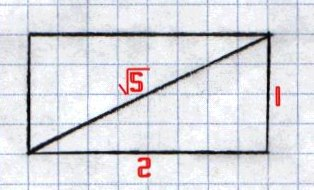The Golden Rectangle also shows the Golden Ratio. A Golden Rectangle is a rectangle with proportions that are two consecutive numbers from the Fibonacci sequence. The example at right, 1, 2, is the most common, but Golden Rectangles are all over the place—take 3 x 5 index cards, as another example.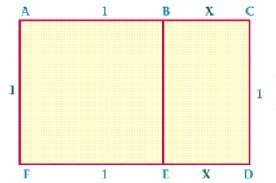In the diagram at left ACDF is a golden rectangle, ABEF is a square, and BCDE is a golden rectangle. The ratio of the length of the longest side to the length of the shortest side of a, golden rectangle is the Golden Ratio.

 The Golden Ratio can be mathematically derived from this relationship by the proportion shown at the right.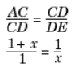If we solve the equation appearing above for x, we'll find that it is the value
 1 + √5 2
and is the Golden Ratio! If you have a Golden Rectangle and you cut a square off it the remaining rectangle will also be a Golden Rectangle. You can keep cutting these squares off and getting smaller and smaller Golden Rectangles.

The Golden Spiral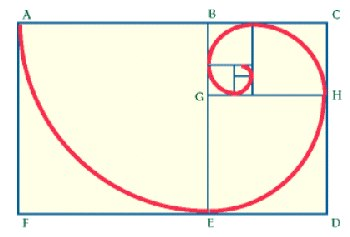The Golden Ratio may also be seen in a spiral. A spiral can be drawn from the diagram above by dividing BCDE into a square and another golden rectangle, and by continuing to separate the golden rectangles into squares and new golden rectangles. The first part of the spiral is drawn with a compass point at B and an arc from A to E; next, the compass point at G and an arc from E to H. Continue in this way until the spiral is completed to your satisfaction. The spiral appears in nature in shells, flower petals, and pine cones.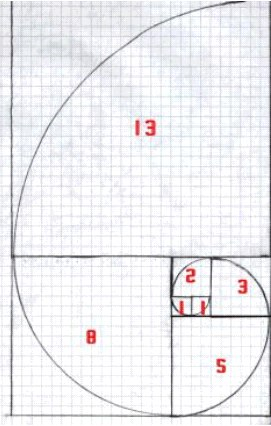The Golden Spiral on the left was created by making adjacent squares of Fibonacci dimensions. An arc is then made across each square. This spiral is called equiangular, because for each quarter turn (90° or π/2), the spiral increases by a factor of Φ. That is, if you take one point, and then a second point one-quarter of a turn away from it, the second point is Φ times farther from the center than the first point. All equiangular, or logarithmic spirals increase by a factor of Φ.

When the golden spiral is graphed on a polar axis, it looks like the image to the right.

 The coordinates of a point, such as A, are written as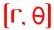. Points can also be written in terms of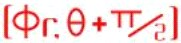.In these instances,and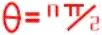. If you combine these two equations, you end up with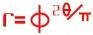.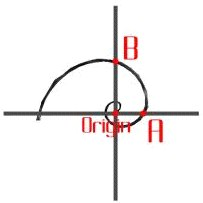And now for the helpful responses to Louis' original question:

Dan Donaldson: You can play with these just a bit to make them fit with fractions, but here is one way to do it. I took the Fibonicci series as: 1, 1, 2, 3, 5, 8, 13, 21, 34. You could go higher, but I don't think it will make much difference in accuracy. Then I added up the last three numbers—13, 21, and 34—to get 68. I took the ratio of the 89 7/8 (rounded to 90) and got 1.32. I took the 1.32 times the last three numbers and got 17.2, 27.8, and 45 as the three distances.

Hoa Dinh: I got 17 3/16", 27 ¾", and 44 15/16". Call them a, b, c, from shortest to longest. Golden Ratio gives: c = 1.618 x b and b = 1.618 x a. Thus a + b + c = a + (1.618 x a) + (1.618 x 1.618 x a) = 5.236 x a = 89 7/8 Solve for a, b, and c.

Sam Simpson: A simple rule of thumb that will always get you very close is to remember the Greek rule of 2–3–5 With a brick having sides that are dimensioned 2, 3, 5 which create faces of 2x3, 2x5 & 3x5 you can stack any number of them, in any combination to build anything.

If you take each of these numbers 2, 3, 5 add them together = 10 then divide them into your height, lets round it to 90 for simplicity's sake, then divide it by ten, you get a factor of nine. 2x9=18 3x9=27 5x9=45 Thus, 18, 27, 45. See how close those are to Dan's numbers?

Dan Donaldson: Actually, that is exactly what I did, the only thing is that Sam used numbers that are a lot easier to work with. 2, 3,and 5 are three of the Fibonacci numbers. I just used slightly bigger ones to do the same thing. Technically, the accuracy will improve as you use bigger numbers, but the difference is negligible, and Sam's numbers are easier to work with.

Warning! If you are not a math geek, you probably do not want to read any further.

Φ, which is the ratio that the Fibonacci series will eventually resolve to is 1.618033989 rounded to nine decimal places. 5/3 will give 1.666666667, which is about 3% high (103.0056648%). The 34/21 division gives 1.619047619, which is about 6/100th of a percent high (100.0626458%). This tells you that the series converges rather rapidly.

In practice, the 2–3–5 is a lot easier to remember, and is accurate enough for most any work, as you will have to play with any dimensions anyway to get something that you can measure.

. . . assembled by Garrett Lambert
with excerpts from The Message Boards